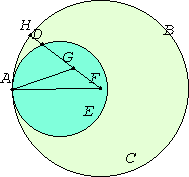Proposition 11

If two circles touch one another internally, and their centers are taken, then the straight line joining their centers, being produced, falls on the point of contact of the circles.
III.1

Let the two circles ABC and ADE touch one another internally at the point A, and let the centers F and G of the circles ABC and ADE be taken.I say that the straight line joined from G to F and produced falls on A.

For suppose it does not, but, if possible, let it fall as FGH. Join AF and AG.

I.20

Then, since the sum of AG and GF is greater than FA, that is, than FH, subtract FG from each, therefore the remainder AG is greater than the remainder GH.

But AG equals GD, therefore GD is also greater than GH, the less greater than the greater, which is impossible.

Therefore the straight line joined from F to G does not fall outside. Therefore it falls on A, the point of contact.

Therefore if two circles touch one another internally, and their centers are taken, then the straight line joining their centers, being produced, falls on the point of contact of the circles.

Q.E.D.

Guide

In order to carry through the proof, in particular so that FA = FH, the circle ABC needs to be the larger circle.

Various conclusions in the proof are based on the figure rather than rigorous deductive reasoning. Camerer and others have suggested ways of filling the gaps.

This proposition is used in III.13.